# 一.综述

Seaborn其实是在matplotlib的基础上进行了更高级的API封装，从而使得作图更加容易，图像也更加美观，本文基于seaborn官方API还有自己的一些理解。

## 1.1.样式控制：axes_style() and set_style()

seaborn提供了5个主题：

• darkgrid 黑色网格（默认）
• whitegrid 白色网格
• dark 黑色背景
• white 白色背景
• ticks 带刻度线import numpy as np
sns.set_style("darkgrid")

def cosplot(flip=1):          #余弦偏移
x = np.linspace(0,14,100)
for i in range(1,7):
plt.plot(x,np.cos(x+i*.5)*(7-i)*flip)
cosplot() # 默认无参数状态，就是删除上方和右方的边框
sns.despine()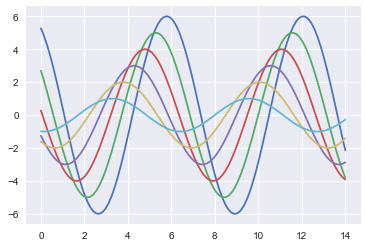## 1.2 .color_palette()创建调色板

### 1.2.1 .分类调色板——分类色板（定性）是在区分没有固定顺序的数据时最好的选择

 1 2 current_palette = sns.color_palette() sns.palplot(current_palette)1 2 current=sns.color_palette('dark') sns.palplot(current)

# 二.连续型单变量的数据可视化

在单变量为分类变量的时候,数据的分析会相对简单,可以通过非常简单的sum,mean等统计变量直接查看自己需要的统计信息基本就能满足我们的需求,非常简单,并不会给我们带来非常大的困难,但是如果单变量为连续变量的时候,数据的可视化就较为重要.

一般最常见的对连续单变量特征进行分析的情况会出现在回归等问题中,这个时候通过可视化的方式可以很快地让我们了解到数据的分布情况以及是否有奇异值的情况.这对我们数据的处理能带来极大的方便.

常用的函数：hist、kdeplot、distplot

首先导入所需要的包import numpy as np
import pandas as pd
import warnings
from scipy import stats,integrate
import matplotlib.pyplot as plt
warnings.filterwarnings('ignore')   #忽略了警告错误的输出
import seaborn as sns
sns.set_style("darkgrid")
sns.set(color_codes=True)  #set( )设置主题，调色板更常用np.random.seed(sum(map(ord, "distributions")))

ord()函数它以一个字符（长度为1的字符串）作为参数，返回对应的ASCII数值，或者Unicode数值.
如果所给的Unicode字符超出了你的Python定义范围，则会引发一个TypeError的异常.
利用np.random.seed()函数设置相同的seed，每次生成的随机数相同。如果不设置seed，则每次会生成不同的随机数.

## 2.1.hist

x=np.random.normal(size=100)
plt.hist(x)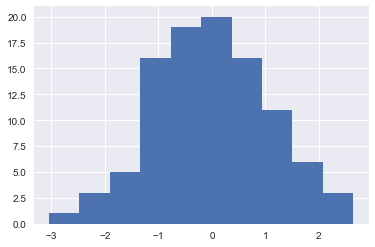## 2.2.kdeplot

seaborn.distplot(a, bins=None, hist=True, kde=True, rug=False, fit=None, hist_kws=None, kde_kws=None, rug_kws=None, fit_kws=None, color=None, vertical=False, norm_hist=False, axlabel=None, label=None, ax=None)
Parameters:

a : Series, 1d-array, or list. #一维数组
bins : argument for matplotlib hist(), or None, optional #设置分桶数
hist : bool, optional #控制是否显示分桶柱子
kde : bool, optional #控制是否显示核密度估计图
rug : bool, optional #控制是否显示观测实例竖线
fit : random variable object, optional #控制拟合的参数分布图形
{hist, kde, rug, fit}_kws : dictionaries, optional
Keyword arguments for underlying plotting functions.
vertical : bool, optional #显示正交控制
If True, oberved values are on y-axis.

sns.kdeplot(x,shade=True)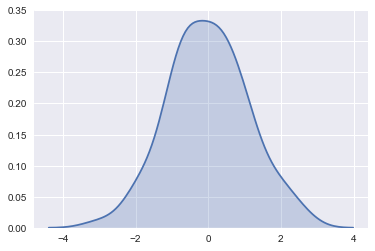KDE的带宽bandwidth（bw）参数控制估计对数据的拟合程度，与直方图中的bin(数据切分数量参数)大小非常相似。 它对应于我们上面绘制的内核的宽度。

sns.kdeplot(x)
sns.kdeplot(x, bw=.2, label="bw: 0.2")
sns.kdeplot(x, bw=2, label="bw: 2")
plt.legend();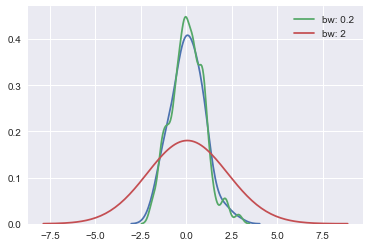## 2.3.displot

上面的两个函数非常实用,但是还有一个非常好的函数,distplot 函数,该函数包含了绝大多数单变量可视化的能力,可以直接使用distplot实现上面两个函数的功能,此外它还可以绘制出其他的近似分布.

默认的distplot能直接绘制出我们需要的直方图以及对应的核密度估计(KDE).

sns.distplot(x)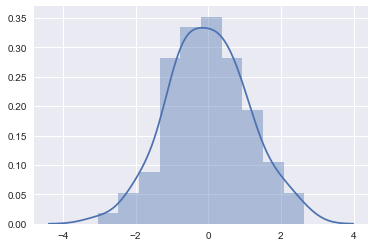当然如果不想看到kde曲线,我们可以直接将kde去掉,如果只想看kde曲线,我们也可以把直方图去掉.

绘制kde曲线一般会比较耗时,所以可以直接将kde设置为False

sns.distplot(x,kde=False,rug=True)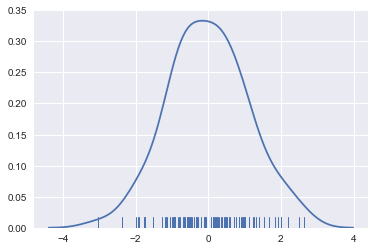当然也可以将直方图省去.仅看kde曲线

sns.distplot(x,hist=False,rug=True)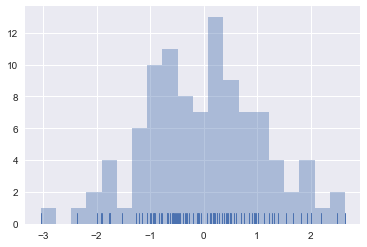y=np.random.gamma(6,size=200)

sns.distplot(y,bins=20,rug=True)

sns.distplot(y,bins=20,kde=False,rug=True,fit=stats.gamma)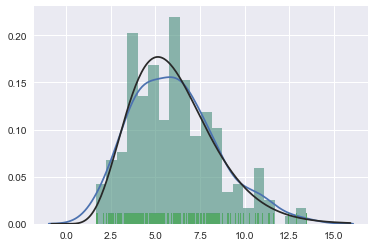# 二.连续(continious)的二元变量特征的数据可视化

上面的数据可视化是基于单变量的,常常用于对连续形式的label(回归问题中较为常见)进行观察处理.实际问题中我们不会仅仅只对数据进行观察,还需要进行预测等,自然而然的,数据之间关系的分析就显得非常重要.此处我们开始对数据之间的关系进行分析,主要是两个连续变量之间的相关性分析.关于涉及分类变量的部分我们会在第后续分进行介绍.

这一节主要介绍如下几个函数matplotlib.pyplot中的scatterplot函数以及seaborn中的jointplot函数.

用多元高斯分布函数生成两个变量x,y.

mean,cov=[0,1],([0.5,1],(1,0.5))
data=np.random.multivariate_normal(mean,cov,size=200)
df=pd.DataFrame(data,columns=['x','y'])
df.head()

## 2.1.Scatterplot函数

这个函数是通用的,尤其是两个变量都是连续型变量的时候,我们希望看看在二维平面上二者之间的关系时必然会先想到散点图函数,当然当数据比较多的时候,建议采样观察[,不然真的很耗时.
通过Scatterplot我们可以很容易的发现一些数据的分布规律,是否有簇的存在等等,在涉及类似于经纬度的问题时,我们经常会通过scatterplot看数据,然后考虑聚类等操作.

plt.scatter(df['x'].values,df['y'].values)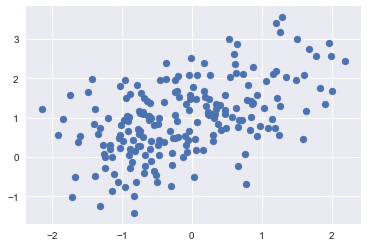## 2.2.Jointplot函数

Scatterplot函数是非常实用的,但是用过seaborn的伙伴肯定都还会知道joinplot这个函数,不仅能方便的绘制散点图,同时还融入了很多其他功能,还可以帮我们直接进行一些简单的模型的拟合(linear regression,etc)

joinplot这个函数显示两个变量之间的双变量（或联合）关系以及每个变量的单变量（或边际）分布和轴。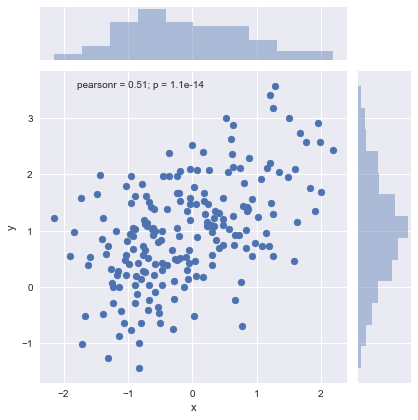sns.jointplot(x='x',y='y',data=df,kind='reg')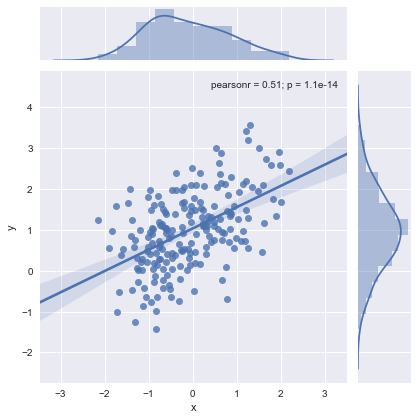Hexbin绘图和KDE绘图,这两个绘图大致可以更加直观的炫酷的看出数据的一个分布情况(例如hex图,越白的地方数据就越少,基本都没有数据在那里).

sns.jointplot(x="x", y="y", data=df,kind ='hex' );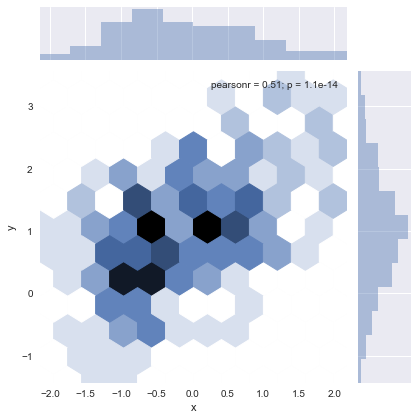with sns.axes_style("white"):
sns.jointplot(x=x, y=y, kind="hex", color="k");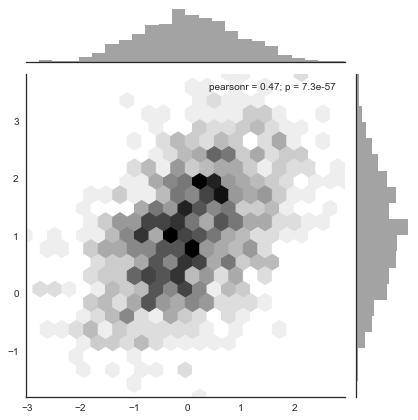sns.jointplot(x="x", y="y", data=df, kind="kde");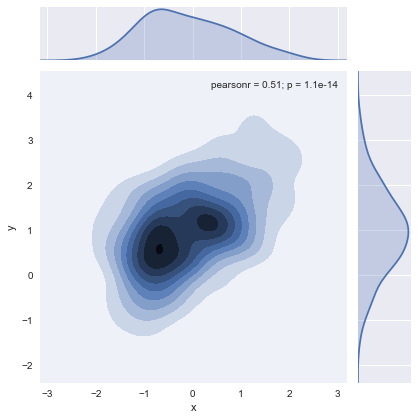f, ax = plt.subplots(figsize=(6, 6))
sns.kdeplot(df.x, df.y, ax=ax)
sns.rugplot(df.x, color="g", ax=ax)
sns.rugplot(df.y, vertical=True, ax=ax);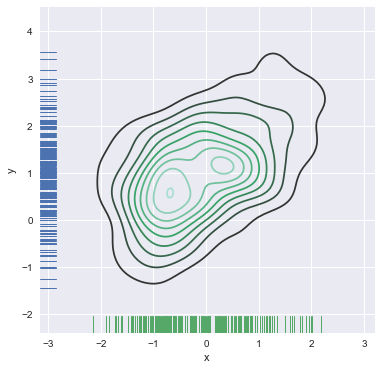f, ax = plt.subplots(figsize=(6, 6))
cmap = sns.cubehelix_palette(as_cmap=True, dark=0, light=1, reverse=True)
sns.kdeplot(df.x, df.y, cmap=cmap, n_levels=60, shade=True);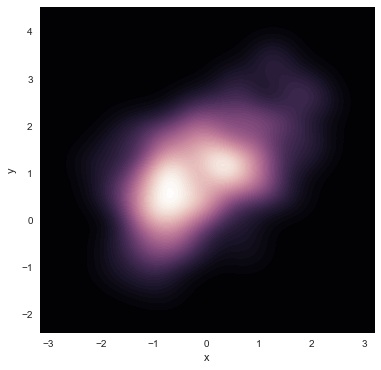plot_joint函数,该绘图函数可以将很多绘图形式放在同一张图表中,更加丰富我们的视图.

g = sns.jointplot(x="x", y="y", data=df, kind="kde", color="m")
g.plot_joint(plt.scatter, c="w", s=30, linewidth=1, marker="+")

g.ax_joint.collections.set_alpha(0)
g.set_axis_labels("$X$", "$Y$");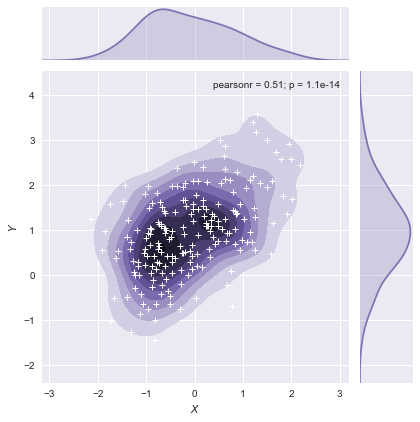## 2.3.pairplot函数

该函数会同时绘制数据中所有特征两两之间的关系图.因为pairplot是建立在pairgrid之上,所以可以将中间的很多函数进行变换,例如下面的kde的例子.

默认对角线histgram，非对角线kdeplot

iris=sns.load_dataset('iris')  #导入经典的鸢尾花数据
sns.pairplot(iris);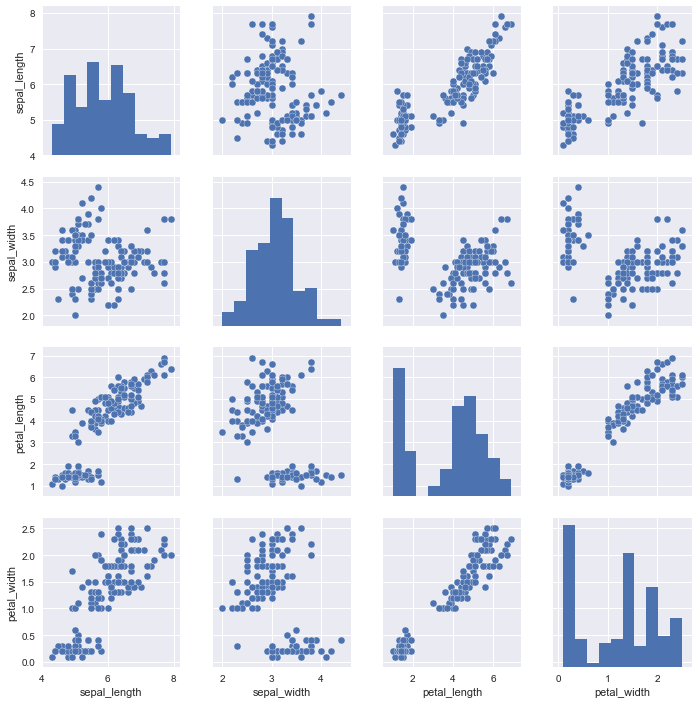g = sns.PairGrid(iris)
g.map_diag(sns.kdeplot)
g.map_offdiag(sns.kdeplot, cmap="Blues_d", n_levels=6);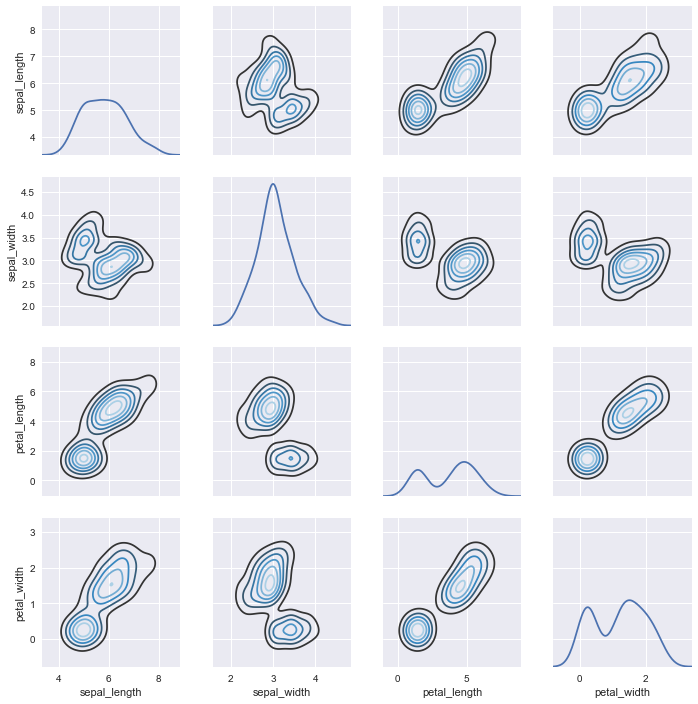g = sns.PairGrid(iris)
g.map_diag(sns.kdeplot)
g.map_offdiag(sns.kdeplot);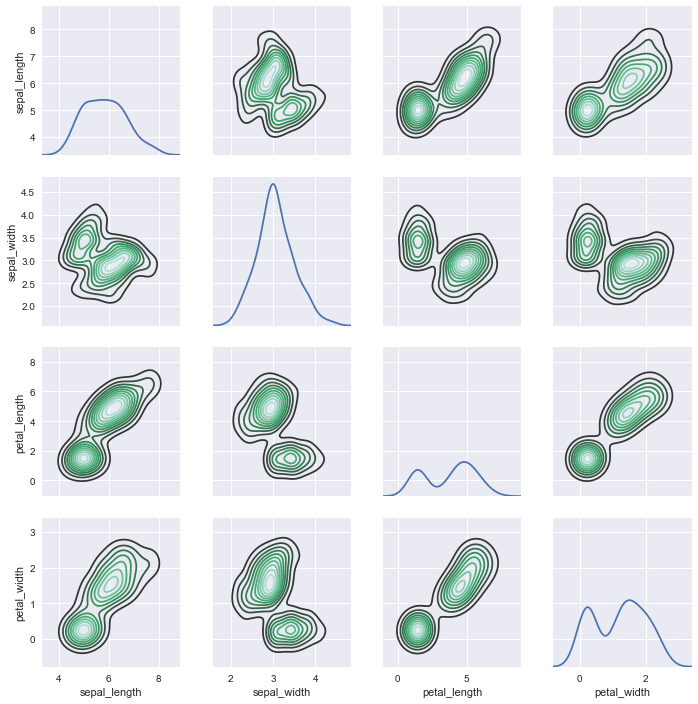05-25
08-22533
03-29152
03-153902
04-081964
05-11113
11-221484
06-234955
12-021万+
04-22486
01-01381
06-19
09-19
10-151558
07-032671
02-025158

### “相关推荐”对你有帮助么？

•非常没帮助
•没帮助
•一般
•有帮助
•非常有帮助被折叠的  条评论 为什么被折叠?到【灌水乐园】发言点击重新获取扫码支付1.余额是钱包充值的虚拟货币，按照1:1的比例进行支付金额的抵扣。
2.余额无法直接购买下载，可以购买VIP、C币套餐、付费专栏及课程。余额充值